# Check Constraints in Oracle

## Check Constraints in Oracle with Examples

In this article, I am going to discuss CHECK Constraints in Oracle with Examples. Please read our previous article where we discussed NOT NULL Constraint in Oracle with Examples. At the end of this article, you will understand everything about the Oracle CHECK Constraints.

##### CHECK Constraint in Oracle:

The Check Constraint in Oracle is used to enforce domain integrity. Domain integrity means the values that are going to store in a table column must be followed by some defined rules such as range, type, and format.

In other words, we can say that Check Constraint ensures the valid entries for a given column value by restricting the type of the value, the format of the data, or the range of possible values.

The CHECK Constraint in Oracle can be applied to single or multiple columns of a table. The CHECK Constraint can be defined while creating a new table or using ALTER TABLE statement for the already existing table.

The CHECK Constraint in Oracle is used to check values with the user-defined conditions before accepting values into a column. The Check Constraints in Oracle can be created at two different levels

1. Column-Level Check Constraints: When we create the check constraints at the column level then they are applied only to that column of the table.
2. Table-level Check Constraints: When we create the check constraints at the table level, then it can be referred from any column(s) within that table.

A table can contain any number of check constraints and will apply to any column data type like integer, character, and decimal, date, etc.

##### Column-Level Check Constraints in Oracle

Let’s understand how to Create Column Level Check constraints with one real-time example. Please have a look at the following CREATE Table Statement.

```CREATE TABLE Employees(
EmployeeID INT CHECK(EmployeeID BETWEEN 100 AND 1000),
NAME VARCHAR(20),
AGE  INT NOT NULL CHECK (AGE >= 18),
DeptID INT CHECK(DeptID > 0 AND DeptID < 100),
SALARY NUMBER(10)
);
```

In the above CREATE Table statement, we have applied three check constraints.

1. The First CHECK Statement is applied on the EmployeeId column which will only allow the values in the range between 100 and 1000.
2. The Second CHECK Constraint is applied on AGE Column which will only allow the values which are greater than equal to 18.
3. The third CHECK Constraint is applied on the DeptID column which will only allow the values if it is greater than 0 and less than 100.
###### Examples:

Now, let us prove the above three statements. Please execute the following two INSERT Statements.

```INSERT INTO Employees values (100, 'Anurag', 20, 1, 20000);
INSERT INTO Employees values (101, 'Mohanty', 25, 2, 25000);```

When you execute the above two SQL INSERT statements, two records are inserted into the Employees table as shown in the below image. This is because the data we inserted satisfied all the Check Constraints.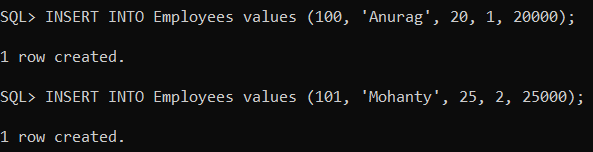Now, try to execute the below INSERT Statement.

`INSERT INTO Employees values (10, 'Sambit', 20, 1, 20000);`

When you try to execute the above SQL INSERT Statement, you will get the error ORA-02290: check constraint (SYSTEM.SYS_C007633) violated as shown in the below image. This is because, here, we trying to INSERT an employee with the EmployeeId value 10 which does not satisfy the CHECK Constraint applied on the EmployeeId column.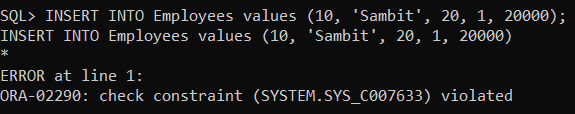Now, try to execute the below INSERT Statement.

`INSERT INTO Employees values (102, 'Sambit', 10, 1, 20000);`

When you try to execute the above SQL INSERT Statement, you will get the error ORA-02290: check constraint (SYSTEM.SYS_C007634) violated as shown in the below image. This is because, here, we trying to INSERT an employee with the AGE value 10 which does not satisfy the CHECK Constraint applied on the AGE column.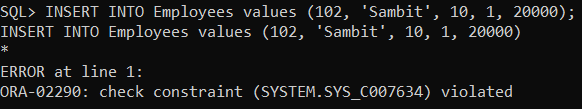Now, try to execute the below INSERT Statement.

`INSERT INTO Employees values (102, 'Sambit', 20, 105, 20000);`

When you try to execute the above SQL INSERT Statement, you will get the error ORA-02290: check constraint (SYSTEM.SYS_C007635) violated as shown in the below image. This is because, here, we trying to INSERT an employee with the DeptID value 105 which does not satisfy the CHECK Constraint applied on the DeptID column.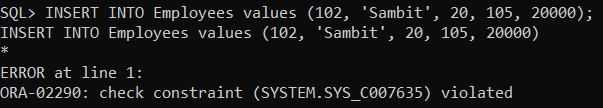##### Table-Level Check Constraints in Oracle:

It is also possible in Oracle to create the CHECK Constraint at the table level. When we create the check constraints at the table level, then that constraint can be referred from any column(s) within that table. Let us understand Table-level Check Constraints with an example. Please have a look at the following CREATE Table statement. Here, we have applied three CHECK Constraints at the table level on the PersonID, AGE, and DeptID columns.

```CREATE TABLE Persons(
PersonID INT,
NAME VARCHAR(20),
AGE  INT,
DeptID INT,
SALARY NUMBER(10),
CONSTRAINT CONSTRAINT_PersonID CHECK(PersonID BETWEEN 100 AND 1000),
CONSTRAINT CONSTRAINT_AGE CHECK(AGE >= 18),
CONSTRAINT CONSTRAINT_DeptID CHECK(DeptID > 0 AND DeptID < 100)
);
```
###### Testing:
```INSERT INTO Persons VALUES (101, 'Anurag', 20, 10, 1000); --Allowed
INSERT INTO Persons VALUES (10, 'Anurag', 20, 10, 1000); -- Now Allowed (Check Constraints for PersonID violates)
INSERT INTO Persons VALUES (102, 'Anurag', 15, 10, 1000); -- Now Allowed (Check Constraints for AGE violates)
INSERT INTO Persons VALUES (102, 'Anurag', 20, 105, 1000); -- Now Allowed (Check Constraints for DeptID violates)
```

When you run the above queries, you will get the following output.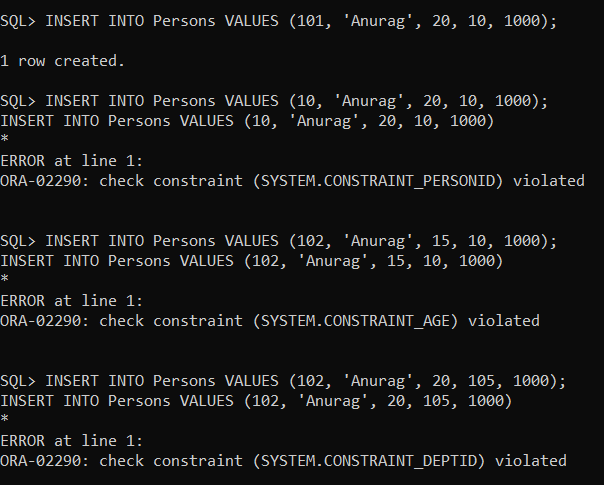##### Composite Check Constraint in Oracle:

While imposing the Constraint at the table level, it is also possible to create the CHECK Constraints on multiple columns in Oracle. Let us understand Composite Check Constraint in Oracle with an example. In the following CREATE TABLE statement, we applied the CHECK Constraint on the AGE and DeptID column.

```CREATE TABLE Person(
PersonID INT,
NAME VARCHAR(20),
AGE  INT,
DeptID INT,
SALARY NUMBER(10),
CONSTRAINT CONSTRAINT_AGE_DepTID CHECK(AGE >= 18 AND DepTID > 0)
);
```
###### Testing:
```INSERT INTO Person VALUES (1, 'Anurag', 20, 10, 1000); --Allowed
INSERT INTO Person VALUES (2, 'Anurag', 15, 20, 1000); -- NOT Allowed
INSERT INTO Person VALUES (3, 'Anurag', 22, 0, 1000); --NOT Allowed
INSERT INTO Person VALUES (1, 'Anurag', 15, 0, 1000); --Not Allowed
```

When you run the above queries, you will get the following output.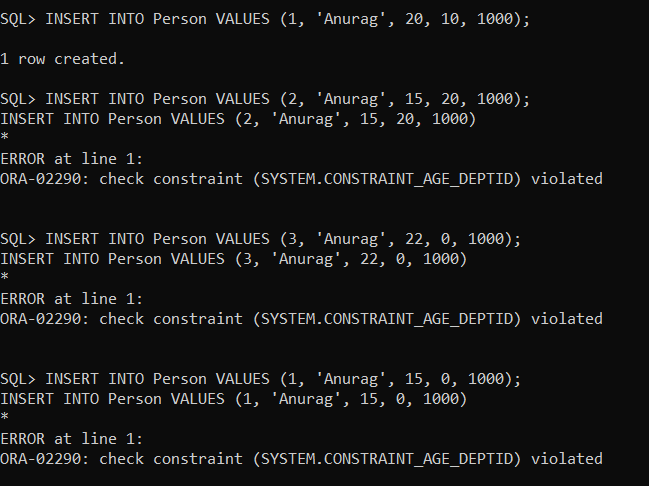In the next article, I am going to discuss the PRIMARY KEY Constraint in Oracle with Examples. Here, in this article, I try to explain CHECK Constraints in Oracle with Examples and I hope you enjoy this CHECK Constraint in Oracle article.